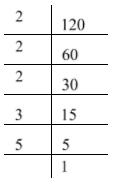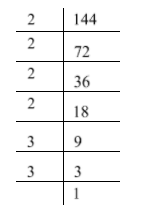QuestionAnswers

# Find the HCF and LCM of $120$ and $144$ by the fundamental theorem of Arithmetic.

Hint:Fundamental theorem of Arithmetic states that every composite number can be factored uniquely as a product of primes. HCF is the highest factor common to two given natural numbers. LCM is the smallest multiple common to two given natural numbers. To find HCF and LCM, first we will find prime factorization of the given natural numbers. Then, observe the common prime factors with smallest power to find HCF and observe all prime factors with greatest power to find LCM.

First we need to find the prime factorization of given numbers $120$ and $144$. These both numbers are even so we can start prime factorization with prime numbers $2$.Therefore, $120 = 2 \times 2 \times 2 \times 3 \times 5 = {2^3} \times {3^1} \times {5^1}$Therefore, $144 = 2 \times 2 \times 2 \times 2 \times 3 \times 3 = {2^4} \times {3^2}$

Now we can see that $2$ and $3$ are common prime factors in the prime factorization of $120$ and
$144$. Also we can see the smallest powers of $2$ and $3$ are $3$ and $1$ respectively.
Therefore, HCF of $120$ and $144$ is the product of ${2^3}$ and ${3^1}$.
That is, HCF of $120$ and $144$ is ${2^3} \times {3^1} = 8 \times 3 = 24$.
Now if we observe prime factorization of both numbers then we can see that there are three prime factors $2,3$ and $5$. Also we can see the greatest powers of $2,3$ and $5$ are $4,2$ and $1$ respectively. Therefore, LCM of $120$ and $144$ is the product of ${2^4},{3^2}$ and ${5^1}$. That is, LCM of $120$ and $144$ is ${2^4} \times {3^2} \times {5^1} = 16 \times 9 \times 5 = 720$.
Hence, HCF and LCM of $120$ and $144$ are $24$ and $720$ respectively.

Note:Fundamental theorem of Arithmetic is also known as unique factorization theorem. In this example, the product of two given numbers $120$ and $144$ is $17,280$. If we take a product of HCF and LCM of these two numbers then we will get the same answer. That is, $24 \times 720 = 17280$. Note that the product of given numbers is equivalent to the product of their HCF and LCM. This is the useful property of HCF and LCM.